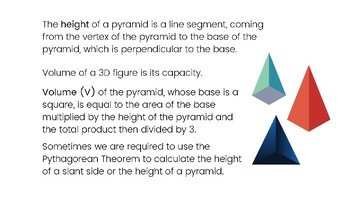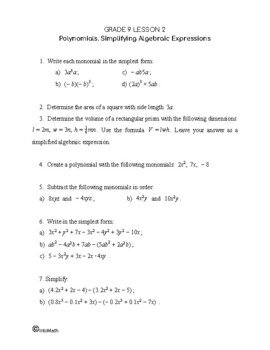CA\$2.00

In this lesson you will learn how to calculate surface area and volume of the given 3D shapes. Volume is how much space the shape has inside (its capacity). Surface Area is the total outside surface of the shape.

In order to calculate the volume of a square-based pyramid, we multiply the area of the base by the height of the pyramid. Sometimes, the height is unknown and needs to be determined using the height of the lateral surface and Pythagorean Theorem